# Relations between cells

It is common for cells in a table to be related to each other. You can use these relations to define math cells and input cells. This allows you to create the table more efficiently and will enable Algebrakit to generate better hints.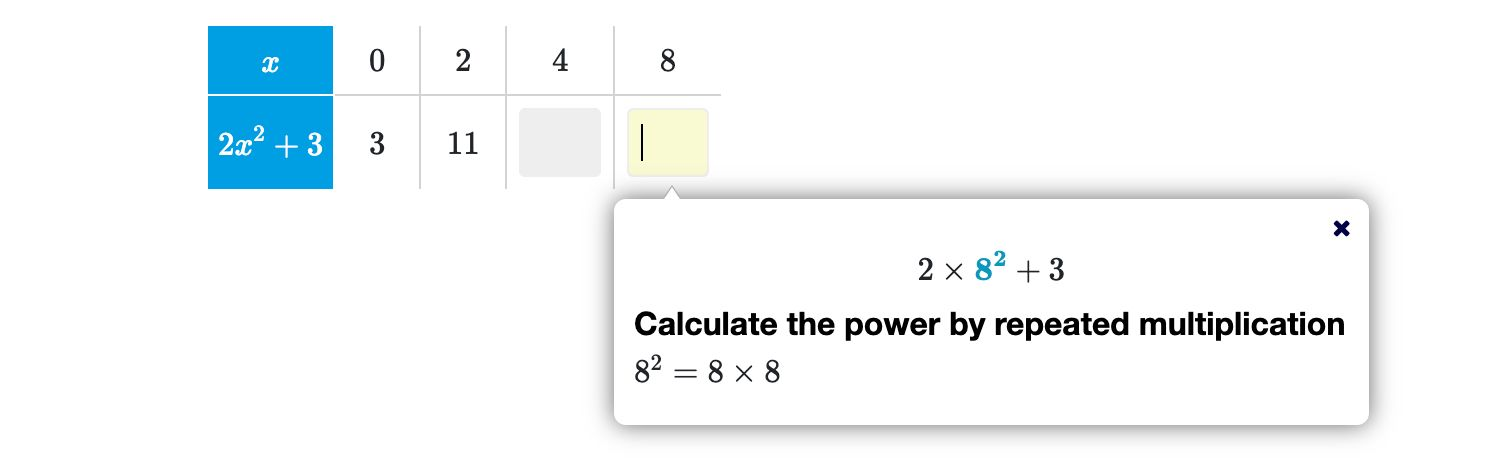Figure: In a function table, the values in the bottom row depend on the values in the top row.

Here is a live example of this table. We will use it as an example to explain relations between cells.

## Referencing cells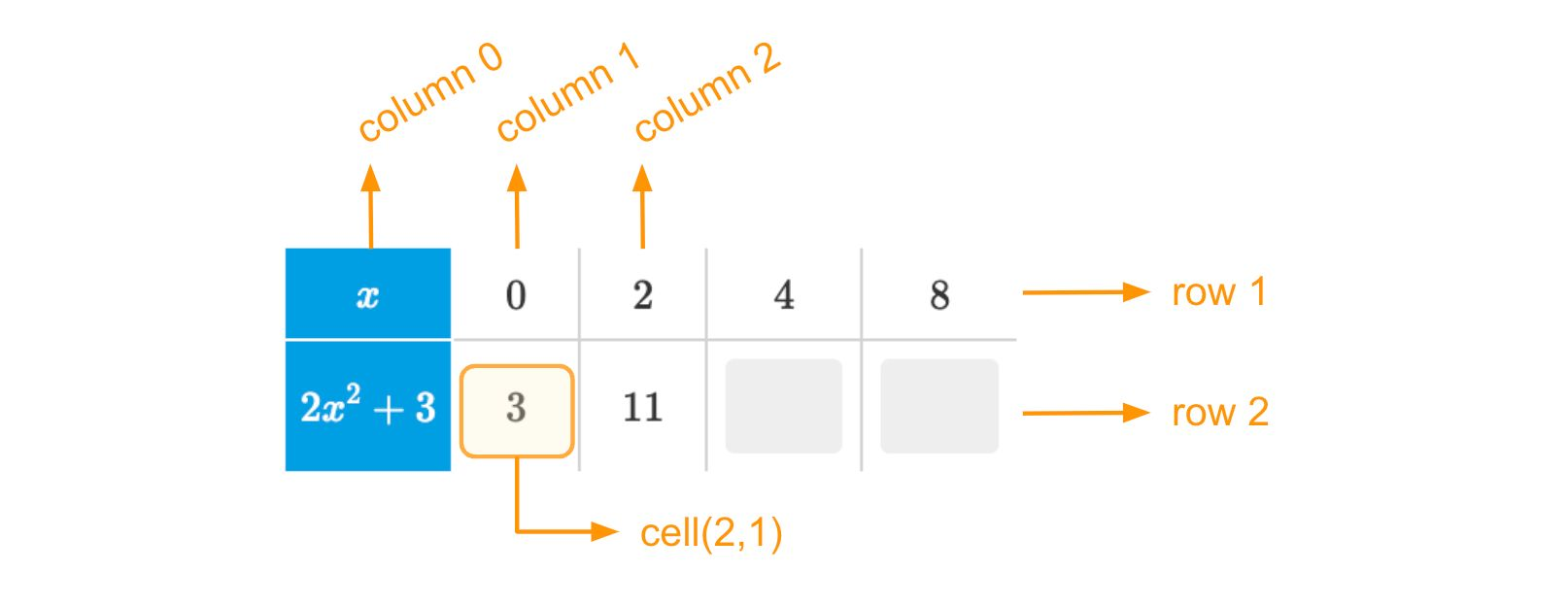Figure: The coordinates of cells in a table.

Each cell in a table has coordinates in the form $cell(row, col)$, where $row$ is the row number, and $col$ is the column number. Row number one is the first non-header row. Column one is the first non-header column. Header cells have row or column numbers zero or lower.

## Defining relations

In our function table, each bottom cell depends on the cell above it through the same mathematical relation. You can use the this definition in each cell, as shown in the figure below. The variables $row$ and $col$ represent the row and column number of the current cell.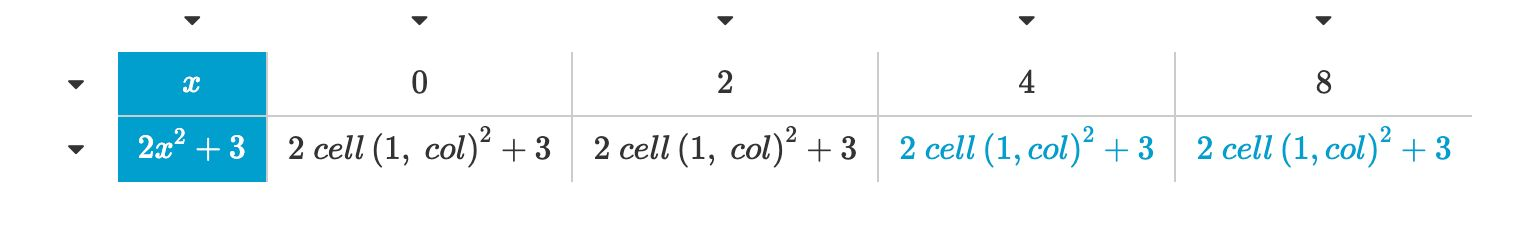Figure: Referencing to cells using 'col' and 'row' variables.

Note that the first two cells in the bottom row are Math cells, and the following are Input cells. Regardless of the type, they all adhere to the same relation.

Note

Algebrakit default shows the expressions $2\cdot 0^2 + 3, 2\cdot 2^2+3$, etc., without evaluating them. To show the results of these expressions, click on a Math cell and check the 'Simplify expression' checkbox below the table.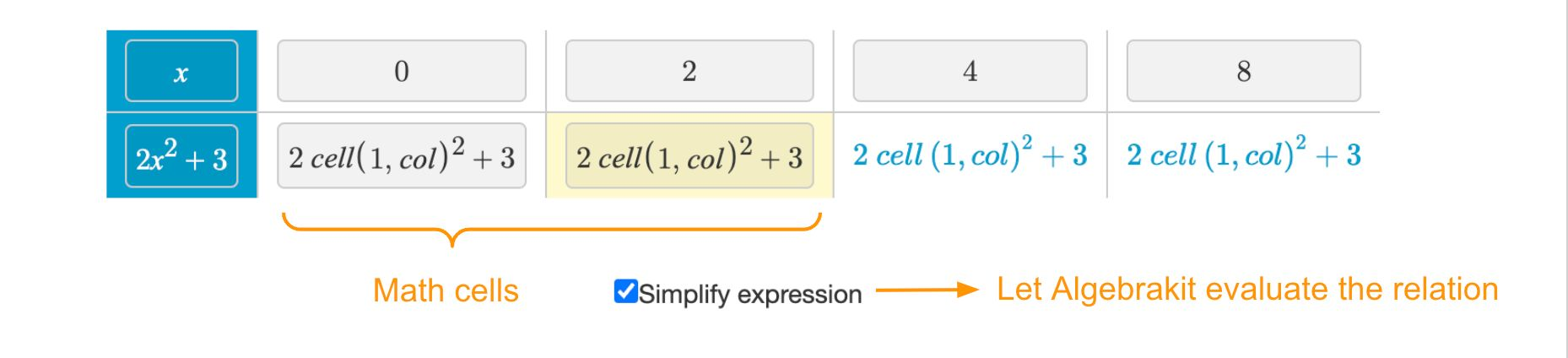Figure: Evaluated expressions in Math cells.

## Use case: Tables with free input

Relations add more flexibility to table questions. For example, you can allow students to decide what values to use.

In this example, the input cells in the top row use evaluation criteria with boolean conditions that allow any integer input. Thanks to the cell references in the bottom cells, Algebrakit can check that the values in those cells are valid.

## Copying cells

If you use relations to define your cells, you will notice that many cells share the same definition. Configuring just one cell and copying it to the others is a convenient and efficient way to set up the table.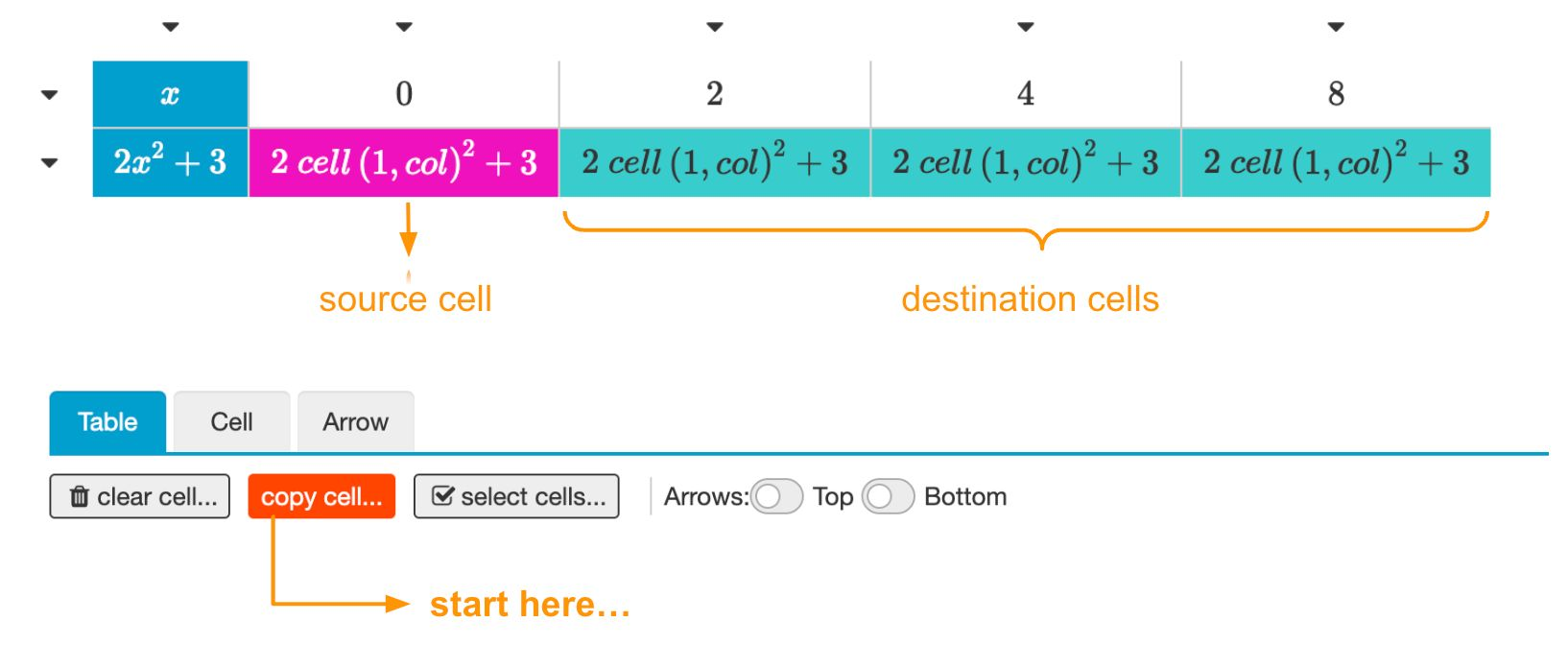Figure: Copying cells.